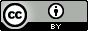# Prolog 匹配 <2>

1. 讨论 Prolog 中的匹配, 解释匹配(match)与相等的不同.
2. 使用 Prolog 搜索的机制解决一些问题.

##Matching

Prolog 中有三种不同的 term, 分别的 constants, variablescomplex terms.

• mia = mia.
• 42 = 42.
• mia = X.
• X = Y.
• friends(john,X) = friends(Y,tom).

1. 如果 term1 和 term2 都是常量, 那么只有当两者是相同的原子或者相同的数字, term1 term2 匹配.
2. 如果 term1 是变量, term2 是任意类型的 term, 那么 term1 和 term2 匹配, term1 会被绑定为 term2.
3. 如果 term1 term2 是 complex term, 那么在下面情况下, 它们会匹配
• 它们含有相同的名字和参数数量.
• 它们对应的参数匹配.
• 变量的绑定是兼容的, 同一个变量不会同时绑定为两个值.
4. 两个 terms 只有在上述 3 个条件之一成立时, 才会匹配.

vertical(line(point(X,Y),point(X,Z))).
horizontal(line(point(X,Y),point(Z,Y))).


?- vertical(line(point(1,1),point(1,3))).
true


?- vertical(line(point(1,1),point(X,4))).
X = 1.


word(abalone,a,b,a,l,o,n,e).
word(abandon,a,b,a,n,d,o,n).
word(enhance,e,n,h,a,n,c,e).
word(anagram,a,n,a,g,r,a,m).
word(connect,c,o,n,n,e,c,t).
word(elegant,e,l,e,g,a,n,t).crosswd(V1,V2,V3,H1,H2,H3):-
word(V1,_,A,_,B,_,C,_),
word(V2,_,D,_,E,_,F,_),
word(V3,_,G,_,H,_,I,_),
word(H1,_,A,_,D,_,G,_),
word(H2,_,B,_,E,_,H,_),
word(H3,_,C,_,F,_,I,_),


?- crosswd(H1,H2,H3,V1,V2,V3).
H1 = abalone,
H2 = anagram,
H3 = connect,
V1 = abandon,
V2 = elegant,
V3 = enhance ;
H1 = abandon,
H2 = elegant,
H3 = enhance,
V1 = abalone,
V2 = anagram,
V3 = connect ;
false.


Prolog 中匹配的能力非常强大, 其实它就是对已经有的条件和数据进行搜索, 尝试所有的答案, 最后给出满足条件的所有结果, 能够极大的降低我们的计算量.### 转载申请### Go 语言设计与实现